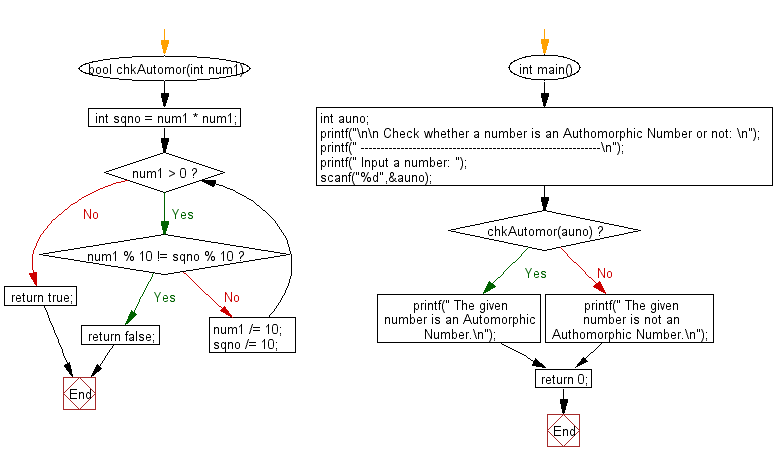﻿ C Program: Check if a number is Authomorphic or not - w3resource# C Exercises: Check if a number is Authomorphic or not

## C Numbers: Exercise-21 with Solution

Write a program in C to check if a number is Authomorphic or not.

Test Data
Input a number: 76

Sample Solution:

C Code:

``````# include <stdio.h>
# include <stdlib.h>
# include <stdbool.h>

bool chkAutomor(int num1)
{
int sqno = num1 * num1;
while (num1 > 0)
{
if (num1 % 10 != sqno % 10)
return false;
num1 /= 10;
sqno /= 10;
}
return true;
}
int main()
{
int auno;
printf("\n\n Check whether a number is an Authomorphic Number or not: \n");
printf(" ------------------------------------------------------------\n");
printf(" Input a number: ");
scanf("%d",&auno);

if( chkAutomor(auno))
printf(" The given number is an Automorphic Number.\n");
else
printf(" The given number is not an Authomorphic Number.\n");
return 0;
}
```
```

Sample Output:

```Input a number: 76
The given number is an Automorphic Number.
```

Pictorial Presentation:Flowchart:C Programming Code Editor:

What is the difficulty level of this exercise?

Test your Programming skills with w3resource's quiz.

﻿

## C Programming: Tips of the Day

Why do C and C++ compilers allow array lengths in function signatures when they're never enforced?

It is a quirk of the syntax for passing arrays to functions.

Actually it is not possible to pass an array in C. If you write syntax that looks like it should pass the array, what actually happens is that a pointer to the first element of the array is passed instead.

Since the pointer does not include any length information, the contents of your [] in the function formal parameter list are actually ignored.

Ref : https://bit.ly/3fhlvdH